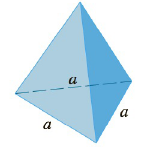Chapter 5.2, Problem 52E

Chapter
Section
Textbook Problem

# Find the volume of the described solid S.A pyramid with height h and base an equilateral triangle with side a (a tetrahedron)To determine

To find:

The volume of a pyramid with height h and base an equilateral triangle with side a.

Explanation

1) Concept:

Definition of volume:

Let S be a solid that lies between x=a and  x=b. If the cross sectional area of S in the plane Px, through x and perpendicular to the x-axis, is  A(x), where A is a continuous function, then the volume of S is

V=limni=1nAxi*x=abAxdx

2) Given:

Height of pyramid is h and basean equilateral triangle with side a.

3) Calculations:

Consider the triangle consisting of two vertices of the base and the center of the base.

This triangle is similar to the corresponding triangle at a height y,

So from the figure,

ab=ΑΒ Α=aΒb.

Also, by similar triangles,

bh=Βh-y Β=bh-yh.

These two equations imply that

Α=a(1-yh)

Since the cross section is an equilateral triangle, it has area

Ay=12·Α·32Α

After substituting the value of Α,

Ay=34·a2

### Still sussing out bartleby?

Check out a sample textbook solution.

See a sample solution

#### The Solution to Your Study Problems

Bartleby provides explanations to thousands of textbook problems written by our experts, many with advanced degrees!

Get Started

#### In Exercises 7-12, solve for y in terms of x. y24x2=2

Calculus: An Applied Approach (MindTap Course List)

#### Evaluate the integral. 18. x2+8x3x3+3x2dx

Single Variable Calculus: Early Transcendentals

#### 2 1 0 does not exist

Study Guide for Stewart's Multivariable Calculus, 8th

#### True or False: The function y = f(x) graphed at the right could be a solution to y = x + y.

Study Guide for Stewart's Single Variable Calculus: Early Transcendentals, 8th